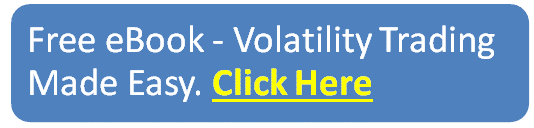blog

# IV Rank vs IV Percentile: Which Should You Use?

#### Options Trading 101 - The Ultimate Beginners Guide To Options

As Seen On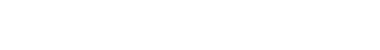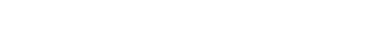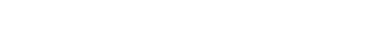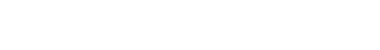by Gavin in Blog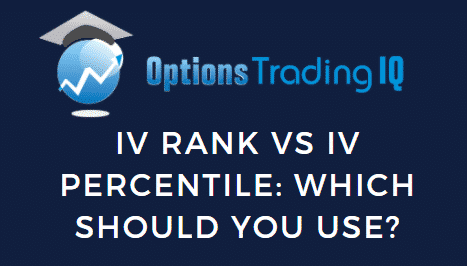When looking at iv rank vs iv percentile, how do we know which is better?

Read on and you will learn when to use each one.

## Introduction

Implied volatility is a very important concept for option traders to understand and is something that always generates a lot of questions among beginners.

When deciding which option strategy to trade, a big part of that decision is based on our opinion of implied volatility. Is implied volatility currently high or low?

This is where IV Rank and IV Percentile come in.

## Implied Volatility Rank (IV Rank) Explained

IV Rank compares a stock’s current level of implied volatility and compares it with the range of implied volatility over a certain time period, usually one year.

You can read about it in detail here, but let’s have a quick refresher.

IV Rank is calculated as follows: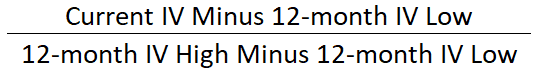Let’s look at an example using Salesforce stock (CRM)

Date: August 31st, 2020

Current IV: 43.80%

12-month Low IV: 18.14%

12-month High IV: 104.58%

This give us the following calculation: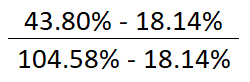The resulting value is 29.69 and that is the IV Rank as of that date.

Pretty easy, right?

This figure of 29.69 that the current level of implied volatility is closer to the low end of the range that has been seen in the last 12 months.

If the IV rank was zero, that would indicate that the current implied volatility was at the very bottom of the 12-month range and an IV rank of 100 would indicate that it was at the very top of the 12-month range.

Let’s no take a look at the implied volatility percentile.

## Implied Volatility Percentile (IV Percentile) Explained

IV percentile indicates the percentage of days with implied volatility closing below the current implied volatility over the period.

Here’s how it is calculated: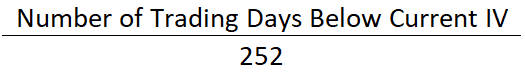We use 252 as the denominator because that is roughly how many trading days there are in a year once you take out weekends and bank holidays.

Let’s look at our Salesforce example again:

Date: August 31st, 2020

Current IV: 43.80%

Trading Days Below Current IV: 214

Which gives us: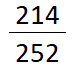Which equals an IV percentile of 85 or 85%. We therefore know that CRM’s implied volatility has been lower than the current level on 85% of trading days in the past year.

Now you understand the difference between the two and how they are calculated, but thankfully you don’t have to make these calculations yourself.

Most brokers will provide this information. Here is the data from Interactive Brokers.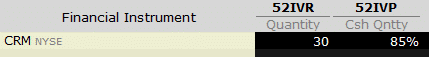## Differences Between IV Rank or IV Percentile?

In this CRM example, we’re seeing quite a big variance in values of IV rank vs IV percentile.

This is because of the massive spike in volatility in March 2020 which is distorting the numbers.

You can see on the chart below (yellow line), that the current level of IV is higher than most days in the last 52 weeks (85% IV Percentile).

BUT… because of the vol spike in March the current value of IV of 45% is a long way below the high of near 95%.

You therefore get a situation where the IV Percentile is high, but the IV Rank is low.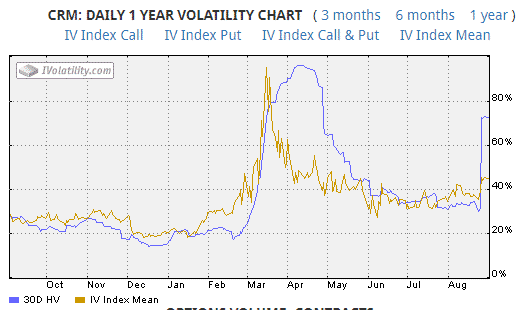## FAQ

### What is IV percentile?

IV percentile indicates the percentage of days with implied volatility closing below the current implied volatility over the period.

### What is IV rank and IV percentile?

IV rank compares the current level of implied volatility to the twelve-month range, whereas IV Percentile looks at the number of trading days below the current level.

### Is high IV percentile good?

A high IV percentile can be good for traders engaged in option selling strategies such as iron condors.

These strategies typically perform better when IV percentile is high.

Implied volatility is like any asset class, we want to buy low and sell high.

Or, in this case, sell high and buy low.

### What is a good IV rank?

For option selling strategies, most traders will look at stocks with and IV rank above 50.

### Is low IV better for options?

Low IV means markets are calm which can be good for options.

However, option premiums will be low which is not ideal for option sellers.

Traders selling options in a low IV environment could suffer large losses if the market flips to high IV.

### How is IV percentile calculated?

IV percentile is typically calculated the number of trading days with IV below the current level, divided by 252.

We use 252 because that is roughly the amount of trading days in a year once weekends and bank holidays are taken out.

### What does high IV mean?

High IV means high implied volatility and this means the market is expecting large futures price movement for the stock.

## Should You Use IV Rank or IV Percentile?

Most trader should realize that you want to sell volatility when it is high via strategies like iron condors and short straddles and buy volatility when it is low via strategies like long straddles and long strangles.

So, which one should we use?

Our CRM example indicated that IV rank is low but IV percentile is high.

Personally, I think that IV rank is slightly flawed because the data gets skewed whenever there is a large spike in volatility.

We see this in our CRM example, when implied volatility is at 43.80% which is higher than the majority of the values seen in the preceding 12 months.

To me IV percentile is the more reliable indicator.

What do you think? Let me know if the comments.